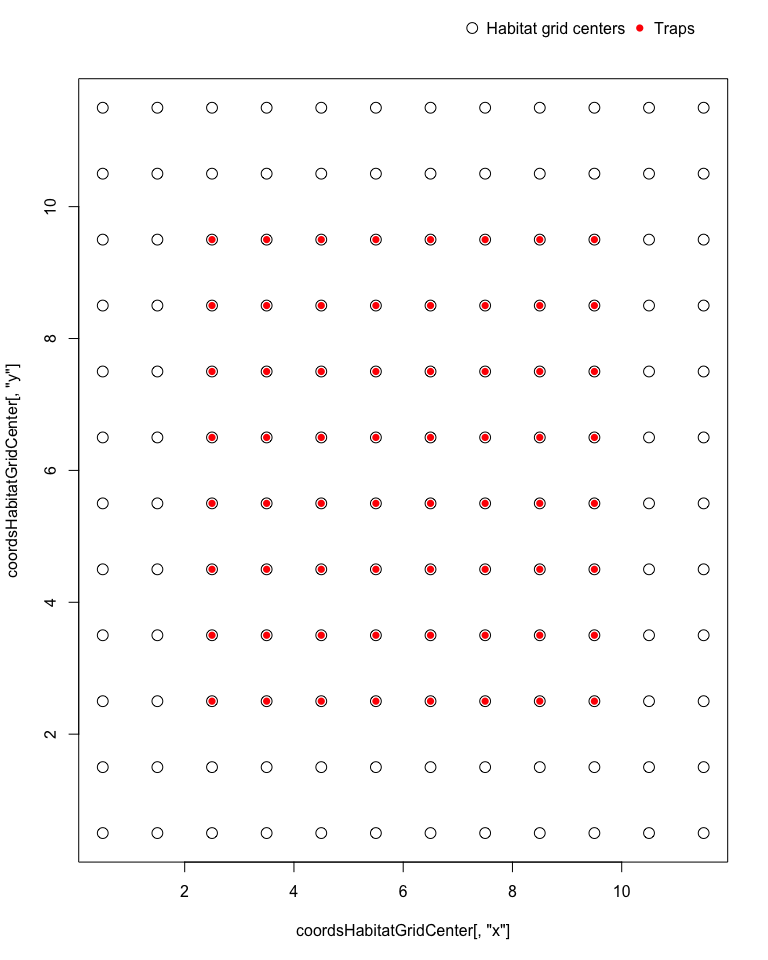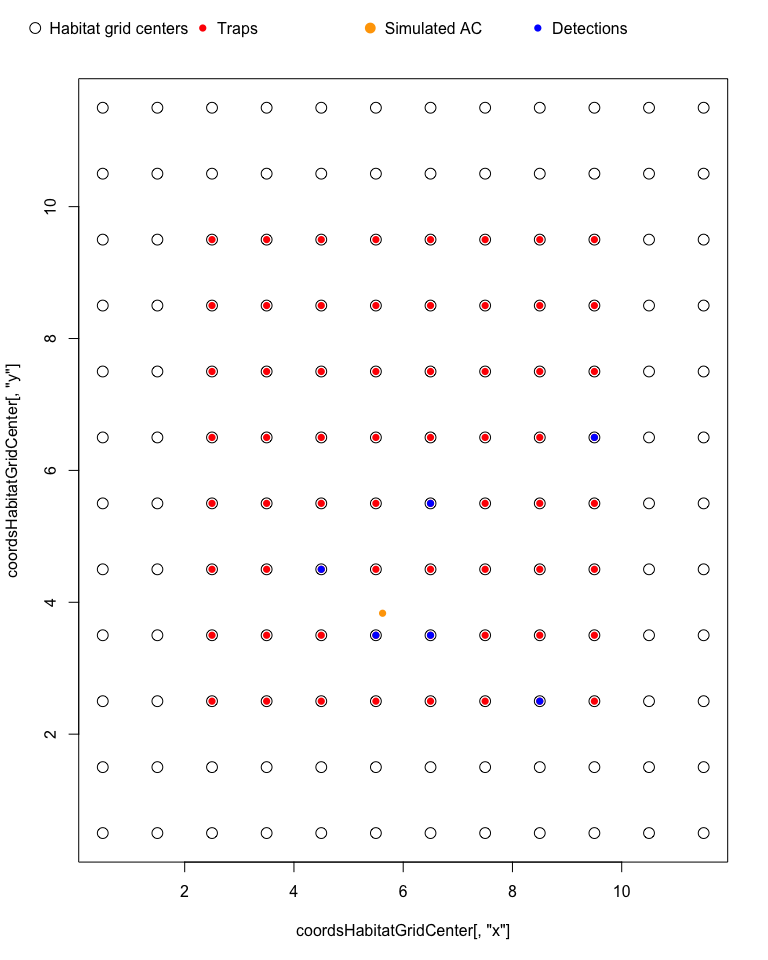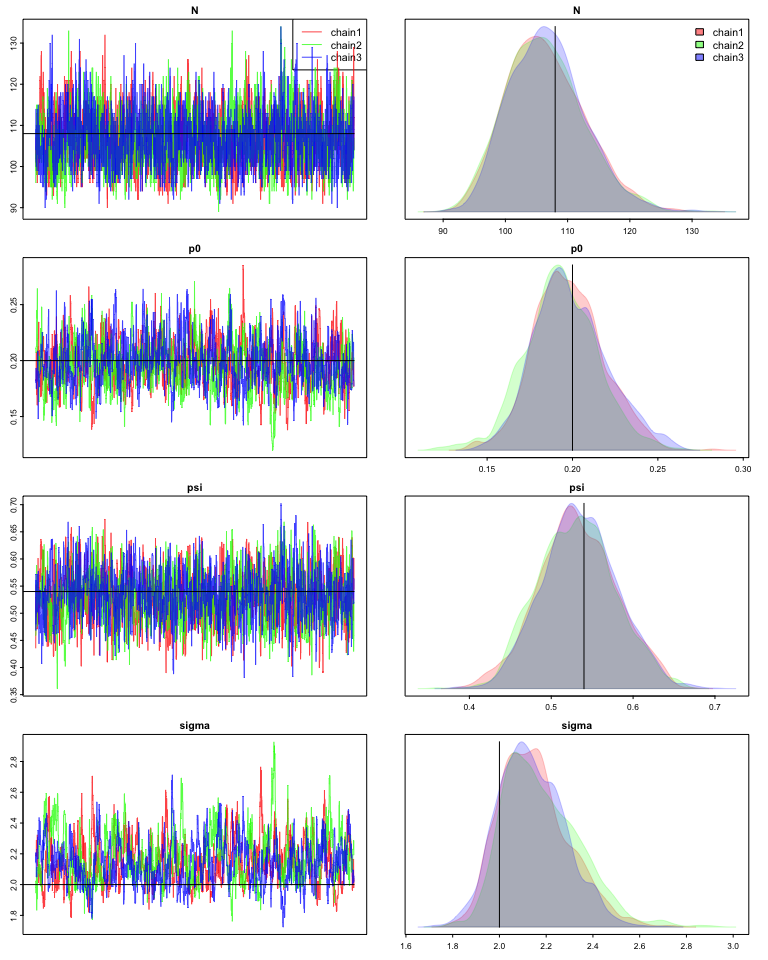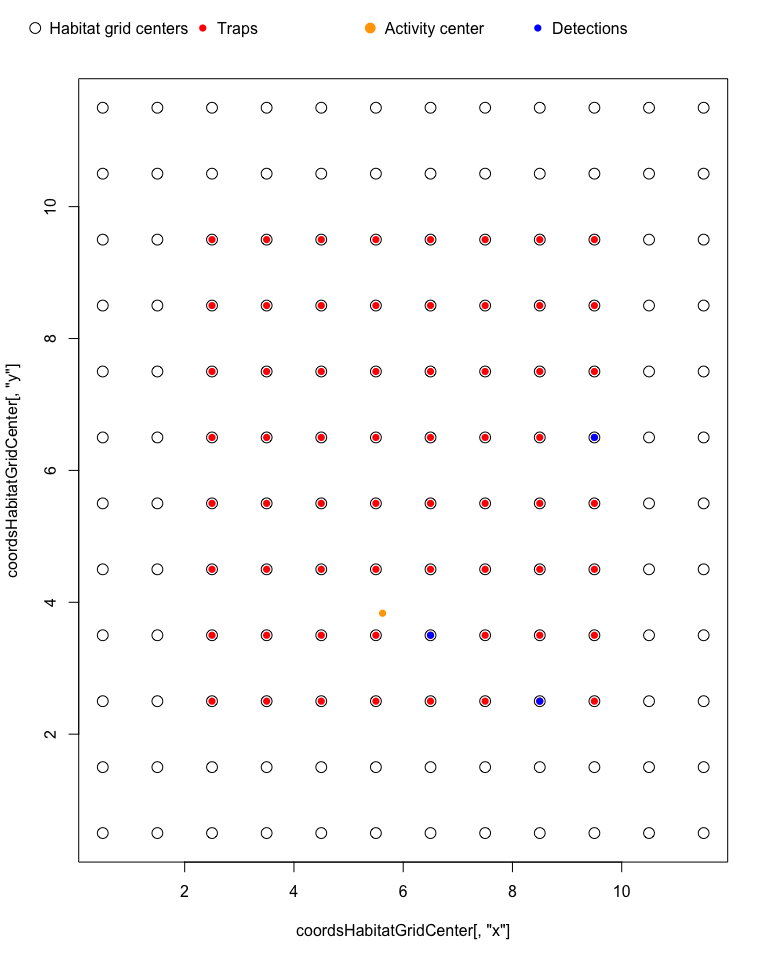# Using nimbleSCR to simulate and fit Bayesian SCR models

#### 2022-11-30

In this vignette, we will use the nimbleSCR (Bischof et al. 2020) package and NIMBLE (de Valpine et al. 2017; NIMBLE Development Team 2020) to simulate and fit an efficient Bayesian SCR model.

# LOAD PACKAGES
library(nimble)
library(nimbleSCR)
library(basicMCMCplots)

## 1. SIMULATE BINOMIAL SCR DATA

### 1.1 Habitat and trapping grid

For this example, we create a $$12*12$$ habitat grid represented by grid cell centers. We center a $$8*8$$ trapping grid leaving a buffer area of 2 units around it.

# CREATE HABITAT GRID
coordsHabitatGridCenter <- cbind(rep(seq(11.5, 0.5, by=-1), 12),
sort(rep(seq(0.5, 11.5, by=1), 12)))
colnames(coordsHabitatGridCenter) <- c("x","y")

# CREATE TRAP GRID
trapCoords <- cbind(rep(seq(2.5, 9.5,by=1),8),
sort(rep(seq(2.5, 9.5,by=1),8)))
colnames(trapCoords) <- c("x","y")

# PLOT CHECK
plot(coordsHabitatGridCenter[,"y"] ~ coordsHabitatGridCenter[,"x"], pch = 1, cex = 1.5) #pch=16)
points(trapCoords[,"y"] ~ trapCoords[,"x"], col="red", pch=16 )
par(xpd=TRUE)
legend(x = 7, y = 13,
legend=c("Habitat grid centers", "Traps"),
pt.cex = c(1.5,1),
horiz = T,
pch=c(1,16),
col=c("black", "red"),
bty = 'n')### 1.2 Rescale coordinates and local evaluation

Here we rescale the trap coordinates to the habitat to allow a fast habitat cell ID lookup and the local evaluation (see Milleret et al. (2019) and Turek et al. (2021) for further details).

ScaledtrapCoords <- scaleCoordsToHabitatGrid(coordsData =  trapCoords,

lengthYCombined <- 1 + trapLocal$numLocalIndicesMax*2 ### 1.5 Create data, constants and inits objects nimConstants <- list(M = M, n.traps = dim(ScaledtrapCoords), y.max = dim(habitatMask), x.max = dim(habitatMask), y.maxDet = dim(trapLocal$habitatGrid),
x.maxDet = dim(trapLocal$habitatGrid), n.cells = dim(trapLocal$localIndices),
maxNBDets = trapLocal$numLocalIndicesMax, trapIndex = trapLocal$localIndices,
nTraps = trapLocal$numLocalIndices, habitatIDDet = trapLocal$habitatGrid,
lengthYCombined = lengthYCombined)

nimData <- list(trapCoords = ScaledtrapCoords,
ones = rep(1, nimConstants$M), lowerCoords = c(min(coordsHabitatGridCenter[,1]) - 0.5, min(coordsHabitatGridCenter[,2]) - 0.5), upperCoords = c(max(coordsHabitatGridCenter[,1]) + 0.5, max(coordsHabitatGridCenter[,2]) + 0.5), trials = rep(1, dim(ScaledtrapCoords)) ) # We set the parameter values as inits nimInits <- list(p0 = p0, psi = psi, sigma = sigma) ### 1.6 Create NIMBLE model model <- nimbleModel( code = modelCode, constants = nimConstants, data = nimData, inits = nimInits, check = F, calculate = F)  ### 1.7 Simulate SCR data from the NIMBLE model We first need to obtain the list of nodes that will be simulated. We used the ‘getDependencies’ function from NIMBLE. Using the ‘simulate’ function from NIMBLE, we will then simulate the activity center (AC) locations (‘sxy’), the state of the individual (‘z’) and SCR observation data (‘y’) given the values we provided for ‘p0’, ‘sigma’ and ‘psi’. # FIRST WE GET THE NODES TO SIMULATE nodesToSim <- model$getDependencies(c("sxy", "z"), self=T)
# THEN WE SIMULATE THOSE NODES
set.seed(100)
model$simulate(nodesToSim, includeData = FALSE) After running ‘simulate’, the simulated data are stored in the ‘model’ object. For example, we can access the simulated ‘z’ and check how many individuals were considered present: N <- sum(model$z)

We have simulated 108 individuals present in the population of which 86 were detected.

Here we also make some plots to check where are located the simulated detections in relation with the simulated location of the activity center for a given individual.

i <- 5
#NUMBER OF DETECTIONS SIMULATED FOR INDIVIDUAL i
model$y[i,1] ##  6 #LET'S PLOT THEM plot(coordsHabitatGridCenter[,"y"] ~ coordsHabitatGridCenter[,"x"], pch = 1, cex = 1.5) #pch=16) points(trapCoords[,"y"] ~ trapCoords[,"x"], col="red", pch=16 ) # PLOT ITS ACTIVITY CETENR points(model$sxy[i, 2] ~ model$sxy[i, 1],col="orange", pch=16) whichdets <- model$y[i, (trapLocal$numLocalIndicesMax + 2):(trapLocal$numLocalIndicesMax+model$y[i, 1] + 1)] points(ScaledtrapCoords[whichdets, "y"] ~ ScaledtrapCoords[whichdets, "x"], col="blue", pch=16) par(xpd=TRUE) legend(x = -1, y = 13, legend=c("Habitat grid centers", "Traps", "Simulated AC", "Detections"), pt.cex = c(1.5,1), horiz = T, pch=c(1, 16, 16, 16), col=c("black", "red", "orange", "blue"), bty = 'n')## 2. RUN MCMC WITH NIMBLE Here, we build the NIMBLE model again using the simulated ‘y’ as data. For simplicity, we used the simulated ‘z’ as initial values. Then we can fit the SCR model with the simulated ‘y’ data set. nimData1 <- nimData nimData1$y <- model$y nimInits1 <- nimInits nimInits1$z <- model$z nimInits1$sxy <- model$sxy # CREATE AND COMPILE THE NIMBLE MODEL model <- nimbleModel( code = modelCode, constants = nimConstants, data = nimData1, inits = nimInits1, check = F, calculate = F) model$calculate()
##  -1963.177
cmodel <- compileNimble(model)
cmodel$calculate() MCMCconf <- configureMCMC(model = model, monitors = c("N", "sigma", "p0","psi"), control = list(reflective = TRUE), thin = 1) ## ===== Monitors ===== ## thin = 1: N, p0, psi, sigma ## ===== Samplers ===== ## binary sampler (200) ## - z[] (200 elements) ## RW sampler (403) ## - psi ## - sigma ## - p0 ## - sxy[] (400 elements) ## Add block sampling of sxy coordinates see Turek et al 2021 for further details MCMCconf$removeSamplers("sxy")
ACnodes <- paste0("sxy[", 1:nimConstants$M, ", 1:2]") for(node in ACnodes) { MCMCconf$addSampler(target = node,
type = "RW_block",
silent = TRUE)
}

MCMC <- buildMCMC(MCMCconf)
cMCMC <- compileNimble(MCMC, project = model)

niter <- 5000
nburnin <- 1000
nchains <- 3

# RUN THE MCMC
MCMCRuntime <- system.time(myNimbleOutput <- runMCMC( mcmc = cMCMC,
niter = niter,
nburnin = nburnin,
nchains = nchains,
samplesAsCodaMCMC = TRUE))
chainsPlot(myNimbleOutput, line = c(N, p0, N/M, sigma))## 3. SIMULATE BINOMIAL SCR DATA WITH TRAP COVARIATES ON $$p_0$$

Now we imagine a scenario where we would like to build a SCR model with trap covariates on baseline detection probability ($$p_0$$). Compared to the model in (1.3), the baseline detection probability ($$p_0$$) is linearly modelled as a function of two covariates on the logistic scale such as:

$$logit(p0Traps{_j}) = logit(p_0) + BetaTraps_1 * trapCovs1_j + BetaTraps_2 * trapCovs2_j$$

### 3.1 Simulate trap covariates

First, we simulate values for two independent trap covariates.

set.seed(1)
trapCovs <- cbind( runif(nimConstants$n.traps,-1, 1), runif(nimConstants$n.traps,-1, 1))

We now add the trap covariates to the data object.

nimData$trapCovs <- trapCovs We choose the simulated betaTraps values. nimInits$betaTraps <- c(-2, 2)

### 3.2 Define model code

Because we are modelling the effect of trap covariates on baseline detection probability ($$p_0$$), this means that we have a $$p0Traps$$ that varies for each trap j. When this is the case, we need to provide the ‘p0Traps’ argument for the ‘dbinomLocal_normal’ function, because the ‘p0’ argument only accepts a scalar.

modelCodeTrap <- nimbleCode({
## priors
psi ~ dunif(0, 1)
sigma ~ dunif(0, 50)
p0 ~ dunif(0, 1)

#trap covariates
betaTraps ~ dunif(-5,5)
betaTraps ~ dunif(-5,5)
p0Traps[1:n.traps] <- ilogit(logit(p0) + betaTraps * trapCovs[1:n.traps, 1] +
betaTraps * trapCovs[1:n.traps, 2])

## loop over individuals
for(i in 1:M) {
## AC coordinates
sxy[i,1] ~ dunif(0, x.max)
sxy[i,2] ~ dunif(0, y.max)
## habitat constraint
ones[i] ~ dHabitatMask( s = sxy[i,1:2],
xmin = lowerCoords,
xmax = upperCoords,
ymin = lowerCoords,
ymax = upperCoords,
habitat = habitat.mx[1:y.max,1:x.max])
z[i] ~ dbern(psi)
## likelihood
y[i, 1:lengthYCombined] ~ dbinomLocal_normal( size = trials[1:n.traps],
p0Traps = p0Traps[1:n.traps],
s = sxy[i,1:2],
sigma = sigma,
trapCoords = trapCoords[1:n.traps,1:2],
localTrapsIndices = trapIndex[1:n.cells,1:maxNBDets],
localTrapsNum = nTraps[1:n.cells],
habitatGrid = habitatIDDet[1:y.maxDet,1:x.maxDet],
indicator = z[i],
lengthYCombined = lengthYCombined)
}
## derived quantity: total population size
N <- sum(z[1:M])
})

### 3.3 Create NIMBLE model

model <- nimbleModel( code = modelCodeTrap,
constants = nimConstants,
data = nimData,
inits = nimInits,
check = F,
calculate = T)  

### 3.4 Simulate SCR from the nimble model

# FIRST WE GET THE NODES TO SIMULATE
nodesToSim <- model$getDependencies(c("sxy", "z"), self=T) # THEN WE SIMULATE THOSE NODES set.seed(100) model$simulate(nodesToSim, includeData = FALSE)
N <- sum(model$z) We have simulated 108 individuals present in the population of which 93 were detected. Here we make some plots to check where are located the detections in relation with the location of activity center. i <- 5 #NUMBER OF DETECTIONS SIMULATED FOR INDIVIDUAL i model$y[i,1]
##  3
#LET'S PLOT THEM
plot(coordsHabitatGridCenter[,"y"] ~ coordsHabitatGridCenter[,"x"], pch = 1, cex = 1.5) #pch=16)
points(trapCoords[,"y"] ~ trapCoords[,"x"], col="red", pch=16 )
# PLOT ITS ACTIVITY CETENR
points(model$sxy[i, 2] ~ model$sxy[i, 1],col="orange", pch=16)

whichdets <- model$y[i, (trapLocal$numLocalIndicesMax + 2):(trapLocal$numLocalIndicesMax+model$y[i, 1] + 1)]
points(ScaledtrapCoords[whichdets, "y"] ~
ScaledtrapCoords[whichdets, "x"], col="blue", pch=16)

par(xpd=TRUE)
legend(x = -1, y = 13,
legend=c("Habitat grid centers", "Traps", "Activity center", "Detections"),
pt.cex = c(1.5,1),
horiz = T,
pch=c(1, 16, 16, 16),
col=c("black", "red", "orange", "blue"),
bty = 'n')## 4. RUN MCMC WITH NIMBLE

nimData1 <- nimData
nimData1$y <- model$y
nimInits1 <- nimInits
nimInits1$z <- model$z
nimInits1$sxy <- model$sxy

# CREATE AND COMPILE THE NIMBLE MODEL
model <- nimbleModel( code = modelCodeTrap,
constants = nimConstants,
data = nimData1,
inits = nimInits1,
check = F,
calculate = F)
model$calculate() ##  -1976.644 cmodel <- compileNimble(model) cmodel$calculate()
MCMCconf <- configureMCMC(model = model,
monitors = c("N", "sigma", "p0","psi", "betaTraps"),
control = list(reflective = TRUE),
thin = 1)
## ===== Monitors =====
## thin = 1: N, betaTraps, p0, psi, sigma
## ===== Samplers =====
## binary sampler (200)
##   - z[]  (200 elements)
## RW sampler (405)
##   - psi
##   - sigma
##   - p0
##   - betaTraps[]  (2 elements)
##   - sxy[]  (400 elements)
## Add block sampling of sxy coordinates see Turek et al 2021 for further details
MCMCconf$removeSamplers("sxy") ACnodes <- paste0("sxy[", 1:nimConstants$M, ", 1:2]")
for(node in ACnodes) {
MCMCconf$addSampler(target = node, type = "RW_block", control = list(adaptScaleOnly = TRUE), silent = TRUE) } MCMC <- buildMCMC(MCMCconf) cMCMC <- compileNimble(MCMC, project = model, resetFunctions = TRUE) # RUN THE MCMC MCMCRuntime <- system.time(myNimbleOutput <- runMCMC( mcmc = cMCMC, niter = niter, nburnin = nburnin, nchains = nchains, samplesAsCodaMCMC = TRUE)) chainsPlot(myNimbleOutput, line = c(N, nimInits$betaTraps, p0, N/M, sigma))